# Start Here: `datascience` Tutorial¶

This is a brief introduction to the functionality in `datascience`. For a complete reference guide, please see Tables (datascience.tables).

For other useful tutorials and examples, see:

## Getting Started¶

The most important functionality in the package is is the `Table` class, which is the structure used to represent columns of data. First, load the class:

```In : from datascience import Table
```

In the IPython notebook, type `Table.` followed by the TAB-key to see a list of members.

Note that for the Data Science 8 class we also import additional packages and settings for all assignments and labs. This is so that plots and other available packages mirror the ones in the textbook more closely. The exact code we use is:

```# HIDDEN

import matplotlib
matplotlib.use('Agg')
from datascience import Table
%matplotlib inline
import matplotlib.pyplot as plt
import numpy as np
plt.style.use('fivethirtyeight')
```

In particular, the lines involving `matplotlib` allow for plotting within the IPython notebook.

## Creating a Table¶

A Table is a sequence of labeled columns of data.

A Table can be constructed from scratch by extending an empty table with columns.

```In : t = Table().with_columns([
...:     'letter', ['a', 'b', 'c', 'z'],
...:     'count',  [  9,   3,   3,   1],
...:     'points', [  1,   2,   2,  10],
...: ])
...:

In : print(t)
letter | count | points
a      | 9     | 1
b      | 3     | 2
c      | 3     | 2
z      | 1     | 10
```

More often, a table is read from a CSV file (or an Excel spreadsheet). Here’s the content of an example file:

```In : cat sample.csv
x,y,z
1,10,100
2,11,101
3,12,102
```

And this is how we load it in as a `Table` using `read_table()`:

```In : Table.read_table('sample.csv')
Out:
x    | y    | z
1    | 10   | 100
2    | 11   | 101
3    | 12   | 102
```

CSVs from URLs are also valid inputs to `read_table()`:

```In : Table.read_table('https://www.inferentialthinking.com/data/sat2014.csv')
Out:
State        | Participation Rate | Critical Reading | Math | Writing | Combined
North Dakota | 2.3                | 612              | 620  | 584     | 1816
Illinois     | 4.6                | 599              | 616  | 587     | 1802
Iowa         | 3.1                | 605              | 611  | 578     | 1794
South Dakota | 2.9                | 604              | 609  | 579     | 1792
Minnesota    | 5.9                | 598              | 610  | 578     | 1786
Michigan     | 3.8                | 593              | 610  | 581     | 1784
Wisconsin    | 3.9                | 596              | 608  | 578     | 1782
Missouri     | 4.2                | 595              | 597  | 579     | 1771
Wyoming      | 3.3                | 590              | 599  | 573     | 1762
Kansas       | 5.3                | 591              | 596  | 566     | 1753
... (41 rows omitted)
```

It’s also possible to add columns from a dictionary, but this option is discouraged because dictionaries do not preserve column order.

```In : t = Table().with_columns({
...:     'letter': ['a', 'b', 'c', 'z'],
...:     'count':  [  9,   3,   3,   1],
...:     'points': [  1,   2,   2,  10],
...: })
...:

In : print(t)
letter | count | points
a      | 9     | 1
b      | 3     | 2
c      | 3     | 2
z      | 1     | 10
```

## Accessing Values¶

To access values of columns in the table, use `column()`, which takes a column label or index and returns an array. Alternatively, `columns()` returns a list of columns (arrays).

```In : t
Out:
letter | count | points
a      | 9     | 1
b      | 3     | 2
c      | 3     | 2
z      | 1     | 10

In : t.column('letter')
Out: array(['a', 'b', 'c', 'z'], dtype='<U1')

In : t.column(1)
Out: array([9, 3, 3, 1])
```

You can use bracket notation as a shorthand for this method:

```In : t['letter'] # This is a shorthand for t.column('letter')
Out: array(['a', 'b', 'c', 'z'], dtype='<U1')

In : t        # This is a shorthand for t.column(1)
Out: array([9, 3, 3, 1])
```

To access values by row, `row()` returns a row by index. Alternatively, `rows()` returns an list-like `Rows` object that contains tuple-like `Row` objects.

```In : t.rows
Out:
Rows(letter | count | points
a      | 9     | 1
b      | 3     | 2
c      | 3     | 2
z      | 1     | 10)

In : t.rows
Out: Row(letter='a', count=9, points=1)

In : t.row(0)
Out: Row(letter='a', count=9, points=1)

In : second = t.rows

In : second
Out: Row(letter='b', count=3, points=2)

In : second
Out: 'b'

In : second
Out: 3
```

To get the number of rows, use `num_rows`.

```In : t.num_rows
Out: 4
```

## Manipulating Data¶

Here are some of the most common operations on data. For the rest, see the reference (Tables (datascience.tables)).

Adding a column with `with_column()`:

```In : t
Out:
letter | count | points
a      | 9     | 1
b      | 3     | 2
c      | 3     | 2
z      | 1     | 10

In : t.with_column('vowel?', ['yes', 'no', 'no', 'no'])
Out:
letter | count | points | vowel?
a      | 9     | 1      | yes
b      | 3     | 2      | no
c      | 3     | 2      | no
z      | 1     | 10     | no

In : t # .with_column returns a new table without modifying the original
Out:
letter | count | points
a      | 9     | 1
b      | 3     | 2
c      | 3     | 2
z      | 1     | 10

In : t.with_column('2 * count', t['count'] * 2) # A simple way to operate on columns
Out:
letter | count | points | 2 * count
a      | 9     | 1      | 18
b      | 3     | 2      | 6
c      | 3     | 2      | 6
z      | 1     | 10     | 2
```

Selecting columns with `select()`:

```In : t.select('letter')
Out:
letter
a
b
c
z

In : t.select(['letter', 'points'])
Out:
letter | points
a      | 1
b      | 2
c      | 2
z      | 10
```

Renaming columns with `relabeled()`:

```In : t
Out:
letter | count | points
a      | 9     | 1
b      | 3     | 2
c      | 3     | 2
z      | 1     | 10

In : t.relabeled('points', 'other name')
Out:
letter | count | other name
a      | 9     | 1
b      | 3     | 2
c      | 3     | 2
z      | 1     | 10

In : t
Out:
letter | count | points
a      | 9     | 1
b      | 3     | 2
c      | 3     | 2
z      | 1     | 10

In : t.relabeled(['letter', 'count', 'points'], ['x', 'y', 'z'])
Out:
x    | y    | z
a    | 9    | 1
b    | 3    | 2
c    | 3    | 2
z    | 1    | 10
```

Selecting out rows by index with `take()` and conditionally with `where()`:

```In : t
Out:
letter | count | points
a      | 9     | 1
b      | 3     | 2
c      | 3     | 2
z      | 1     | 10

In : t.take(2) # the third row
Out:
letter | count | points
c      | 3     | 2

In : t.take[0:2] # the first and second rows
Out:
letter | count | points
a      | 9     | 1
b      | 3     | 2
```
```In : t.where('points', 2) # rows where points == 2
Out:
letter | count | points
b      | 3     | 2
c      | 3     | 2

In : t.where(t['count'] < 8) # rows where count < 8
Out:
letter | count | points
b      | 3     | 2
c      | 3     | 2
z      | 1     | 10

In : t['count'] < 8 # .where actually takes in an array of booleans
Out: array([False,  True,  True,  True])

In : t.where([False, True, True, True]) # same as the last line
Out:
letter | count | points
b      | 3     | 2
c      | 3     | 2
z      | 1     | 10
```

Operate on table data with `sort()`, `group()`, and `pivot()`

```In : t
Out:
letter | count | points
a      | 9     | 1
b      | 3     | 2
c      | 3     | 2
z      | 1     | 10

In : t.sort('count')
Out:
letter | count | points
z      | 1     | 10
b      | 3     | 2
c      | 3     | 2
a      | 9     | 1

In : t.sort('letter', descending = True)
Out:
letter | count | points
z      | 1     | 10
c      | 3     | 2
b      | 3     | 2
a      | 9     | 1
```
```# You may pass a reducing function into the collect arg
# Note the renaming of the points column because of the collect arg
In : t.select(['count', 'points']).group('count', collect=sum)
Out:
count | points sum
1     | 10
3     | 4
9     | 1
```
```In : other_table = Table().with_columns([
....:     'mar_status',  ['married', 'married', 'partner', 'partner', 'married'],
....:     'empl_status', ['Working as paid', 'Working as paid', 'Not working',
....:                     'Not working', 'Not working'],
....:     'count',       [1, 1, 1, 1, 1]])
....:

In : other_table
Out:
mar_status | empl_status     | count
married    | Working as paid | 1
married    | Working as paid | 1
partner    | Not working     | 1
partner    | Not working     | 1
married    | Not working     | 1

In : other_table.pivot('mar_status', 'empl_status', 'count', collect=sum)
Out:
empl_status     | married | partner
Not working     | 1       | 2
Working as paid | 2       | 0
```

## Visualizing Data¶

We’ll start with some data drawn at random from two normal distributions:

```In : normal_data = Table().with_columns([
....:     'data1', np.random.normal(loc = 1, scale = 2, size = 100),
....:     'data2', np.random.normal(loc = 4, scale = 3, size = 100)])
....:

In : normal_data
Out:
data1      | data2
-0.0971878 | 4.46524
1.16728    | 4.62078
-0.272761  | 3.21061
0.35794    | 4.29068
1.63212    | 5.99984
-0.0435978 | 4.17347
2.89774    | 1.36874
-1.0725    | 6.23875
1.97047    | 1.25073
-0.670396  | 5.34519
... (90 rows omitted)
```

Draw histograms with `hist()`:

```In : normal_data.hist()
```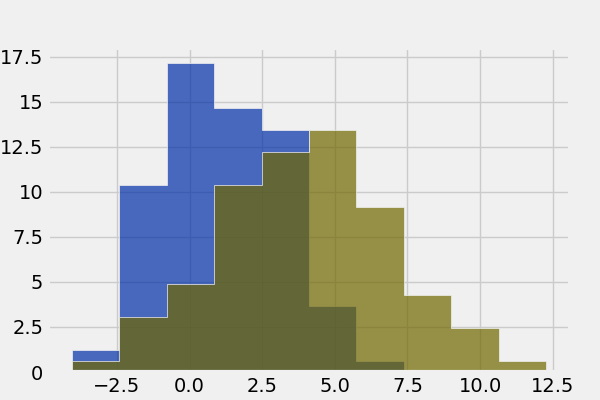```In : normal_data.hist(bins = range(-5, 10))
```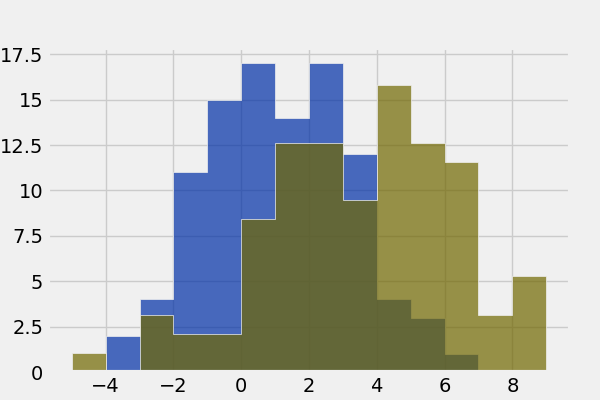```In : normal_data.hist(bins = range(-5, 10), overlay = True)
```If we treat the `normal_data` table as a set of x-y points, we can `plot()` and `scatter()`:

```In : normal_data.sort('data1').plot('data1') # Sort first to make plot nicer
```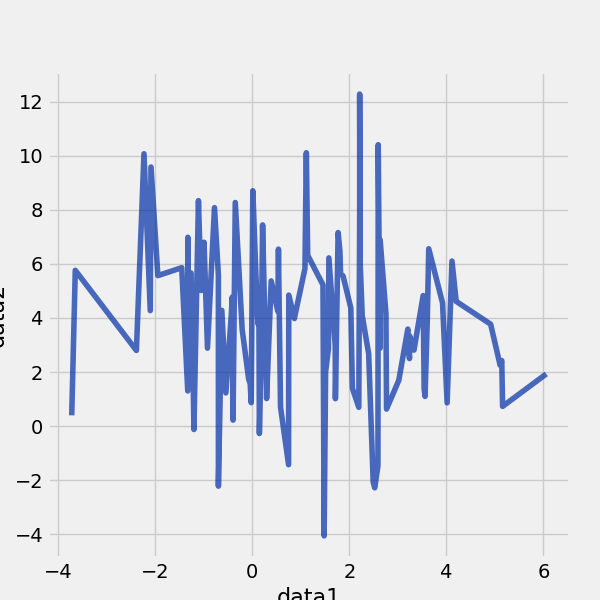```In : normal_data.scatter('data1')
```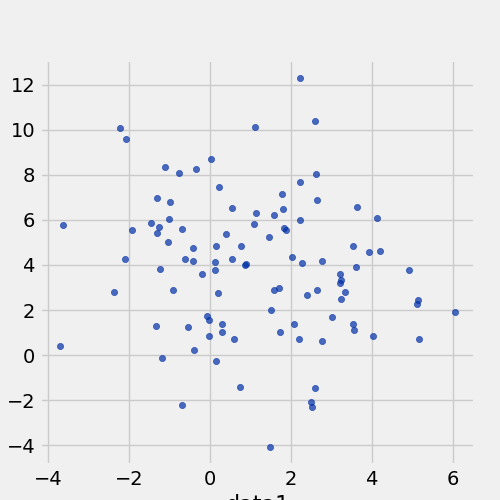```In : normal_data.scatter('data1', fit_line = True)
```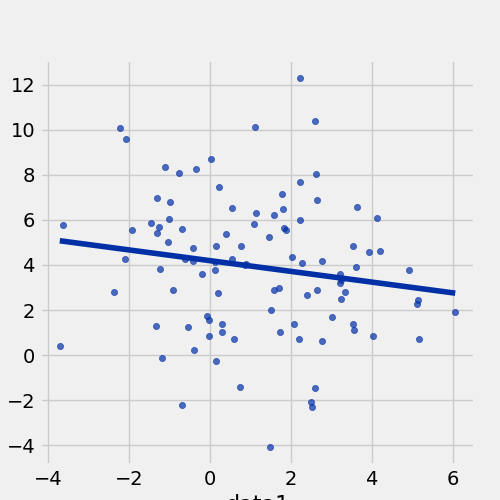Use `barh()` to display categorical data.

```In : t
Out:
letter | count | points
a      | 9     | 1
b      | 3     | 2
c      | 3     | 2
z      | 1     | 10

In : t.barh('letter')
```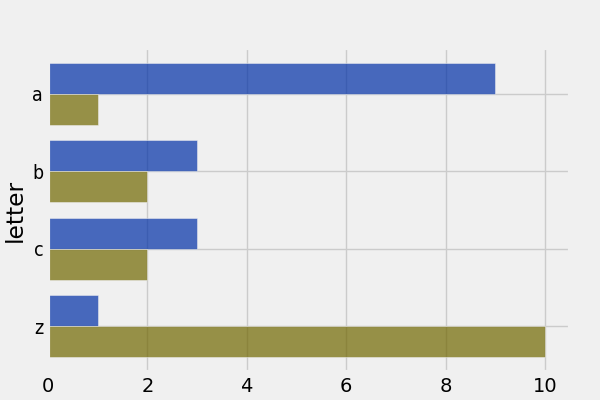## Exporting¶

Exporting to CSV is the most common operation and can be done by first converting to a pandas dataframe with `to_df()`:

```In : normal_data
Out:
data1      | data2
-0.0971878 | 4.46524
1.16728    | 4.62078
-0.272761  | 3.21061
0.35794    | 4.29068
1.63212    | 5.99984
-0.0435978 | 4.17347
2.89774    | 1.36874
-1.0725    | 6.23875
1.97047    | 1.25073
-0.670396  | 5.34519
... (90 rows omitted)

# index = False prevents row numbers from appearing in the resulting CSV
In : normal_data.to_df().to_csv('normal_data.csv', index = False)
```

## An Example¶

We’ll recreate the steps in Chapter 12 of the textbook to see if there is a significant difference in birth weights between smokers and non-smokers using a bootstrap test.

For more examples, check out the TableDemos repo.

From the text:

The table `baby` contains data on a random sample of 1,174 mothers and their newborn babies. The column `Birth Weight` contains the birth weight of the baby, in ounces; `Gestational Days` is the number of gestational days, that is, the number of days the baby was in the womb. There is also data on maternal age, maternal height, maternal pregnancy weight, and whether or not the mother was a smoker.

```In : baby = Table.read_table('https://www.inferentialthinking.com/data/baby.csv')

In : baby # Let's take a peek at the table
Out:
Birth Weight | Gestational Days | Maternal Age | Maternal Height | Maternal Pregnancy Weight | Maternal Smoker
120          | 284              | 27           | 62              | 100                       | False
113          | 282              | 33           | 64              | 135                       | False
128          | 279              | 28           | 64              | 115                       | True
108          | 282              | 23           | 67              | 125                       | True
136          | 286              | 25           | 62              | 93                        | False
138          | 244              | 33           | 62              | 178                       | False
132          | 245              | 23           | 65              | 140                       | False
120          | 289              | 25           | 62              | 125                       | False
143          | 299              | 30           | 66              | 136                       | True
140          | 351              | 27           | 68              | 120                       | False
... (1164 rows omitted)

# Select out columns we want.
In : smoker_and_wt = baby.select(['Maternal Smoker', 'Birth Weight'])

In : smoker_and_wt
Out:
Maternal Smoker | Birth Weight
False           | 120
False           | 113
True            | 128
True            | 108
False           | 136
False           | 138
False           | 132
False           | 120
True            | 143
False           | 140
... (1164 rows omitted)
```

Let’s compare the number of smokers to non-smokers.

```In : smoker_and_wt.select('Maternal Smoker').group('Maternal Smoker')
Out:
Maternal Smoker | count
False           | 715
True            | 459
```

We can also compare the distribution of birthweights between smokers and non-smokers.

```# Non smokers
# We do this by grabbing the rows that correspond to mothers that don't
# smoke, then plotting a histogram of just the birthweights.
In : smoker_and_wt.where('Maternal Smoker', 0).select('Birth Weight').hist()

# Smokers
In : smoker_and_wt.where('Maternal Smoker', 1).select('Birth Weight').hist()
```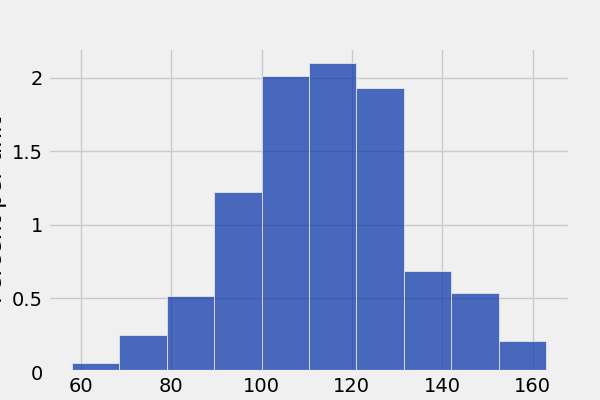What’s the difference in mean birth weight of the two categories?

```In : nonsmoking_mean = smoker_and_wt.where('Maternal Smoker', 0).column('Birth Weight').mean()

In : smoking_mean = smoker_and_wt.where('Maternal Smoker', 1).column('Birth Weight').mean()

In : observed_diff = nonsmoking_mean - smoking_mean

In : observed_diff
Out: 9.266142572024918
```

Let’s do the bootstrap test on the two categories.

```In : num_nonsmokers = smoker_and_wt.where('Maternal Smoker', 0).num_rows

In : def bootstrap_once():
....:     """
....:     Computes one bootstrapped difference in means.
....:     The table.sample method lets us take random samples.
....:     We then split according to the number of nonsmokers in the original sample.
....:     """
....:     resample = smoker_and_wt.sample(with_replacement = True)
....:     bootstrap_diff = resample.column('Birth Weight')[:num_nonsmokers].mean() - \
....:         resample.column('Birth Weight')[num_nonsmokers:].mean()
....:     return bootstrap_diff
....:

In : repetitions = 1000

In : bootstrapped_diff_means = np.array(
....:     [ bootstrap_once() for _ in range(repetitions) ])
....:

In : bootstrapped_diff_means[:10]
Out:
array([ 0.81346801,  0.12834834,  1.4426741 , -0.05151972,  0.36812469,
-1.36601917, -0.38138245, -0.22572025,  1.64743361, -0.00931792])

In : num_diffs_greater = (abs(bootstrapped_diff_means) > abs(observed_diff)).sum()

In : p_value = num_diffs_greater / len(bootstrapped_diff_means)

In : p_value
Out: 0.0
```

To come.